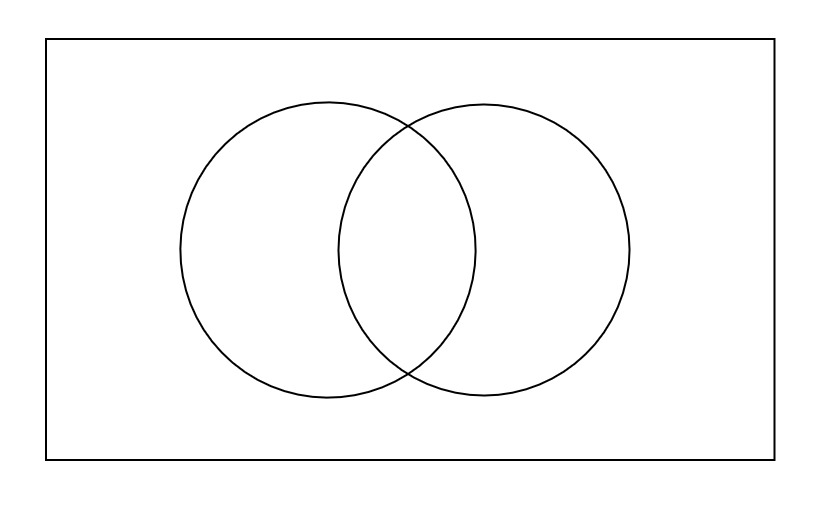# 2.3 Using Venn Diagrams to Calculate Probabilities

Last updated: March 8th, 2020

# Using Venn Diagrams to Calculate Probabilities¶

In the next few videos we will be discussing the use of different tools to calculate probabilities. For these, you will need to have some previous knowledge.

For this video, you will need to know about Venn Diagrams (if you don't please check here).

Now imagine the following experiment: We are picking an integer between $1$ and $10$, and we are interested in the probability of it being odd or prime but not both.

Since the probability of picking each number is the same, this is an experiment with equally likely results, and therefore we can use the formula $P(E)=\dfrac{|E|}{|\Omega|}$.

For that, we could list all the elements in $\Omega$, and all the elements in our event $E$ and then divide those quantities.In an office there are $230$ employees. $85$ of them like tea, $132$ like coffee and $46$ like both tea and coffee. If we pick an employee at random, what will be the probability of they not liking neither tea nor coffee?